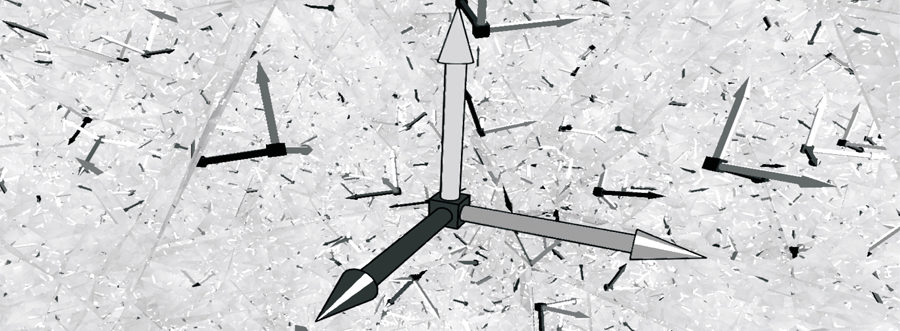# 0 = ∞ ?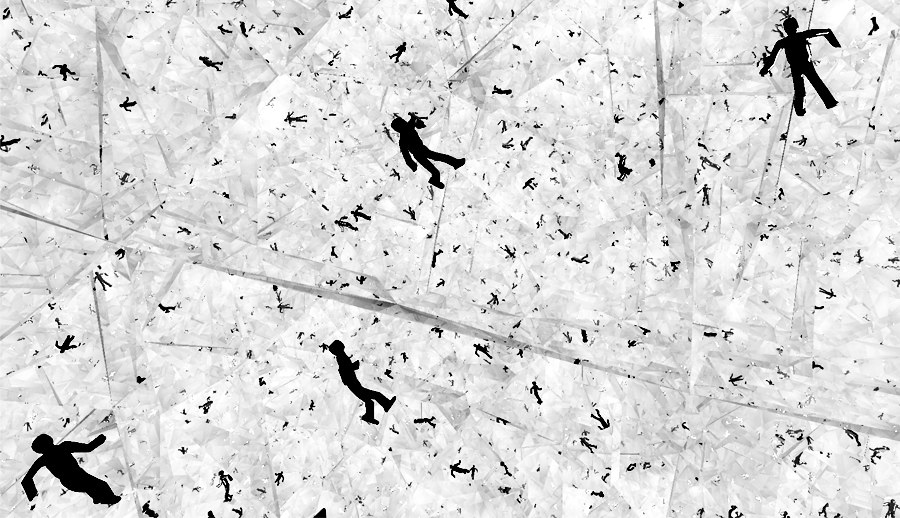• Project team: Hrvoje Čop, Miro Roman, Luka Vlahović
• Year: 09/2004
• Location: space
• Area: 250 m²
• Type: research

### introduction

One's perception of thier surroundings is relative to their own position. Human beings are able to understand four dimensions (length,width, height and time), and perceive those dimensions through their senses. It is possible to calculate more of them mathematically, but not to comprehend nor feel more than those four.

### observation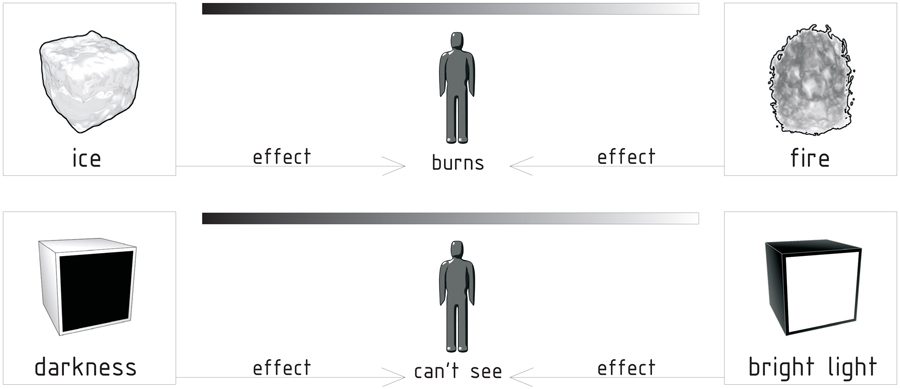### hypothesis

Extremes have the same effect on humans. The examples of effects of two opposite elements, like the ones given above, suggest that the same rule could be applied in the following situation: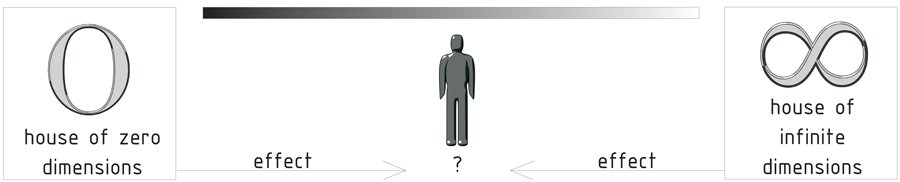Following this assumption, and considering the fact that humans cannot understand more than four dimensions, IF ONE COULD DESIGN A HOUSE OF ZERO DIMENSIONS, IT WOULD CREATE AN ILLUSION OF A HOUSE WITH AN INFINITE NUMBER OF DIMENSIONS.

### house

The house is designed in such a way to negate the four comprehensible dimensions, and thus becoming, in effect, a house of zero dimensions.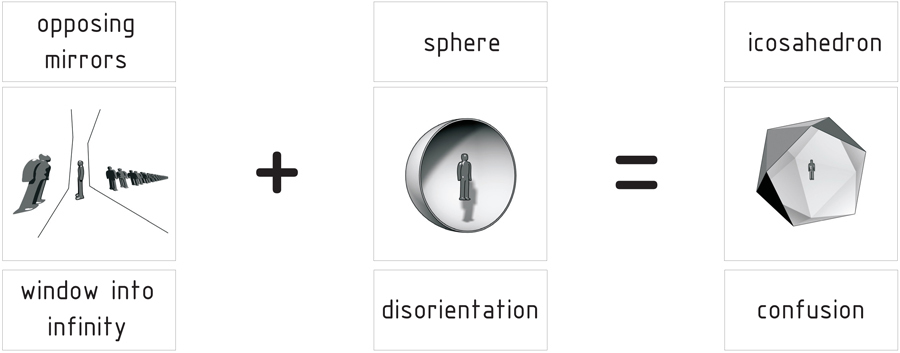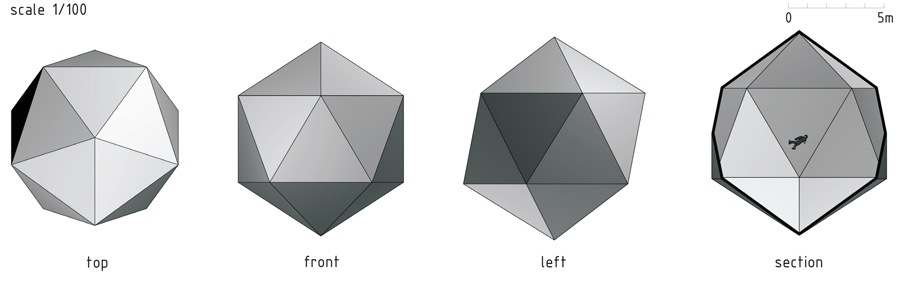The house has the shape of an icosahedron. It is the result of combining a sphere with a system of opposing mirrors. Its surface is 250 m2. Its radius is 4.9 m. It is positioned in universe, where weightlessness works with the mirrors to make impossible for one to understand the space within, nor to determine their position and time in the space. Because one does not have a sense of volume, they are disoriented. They see themselves simultaneously reflected from an infinite number of angles. Since time is always perceived in relation to some fixed event, their existence being the only absolute thing, there is nothing else they could use as a reference point for determining time.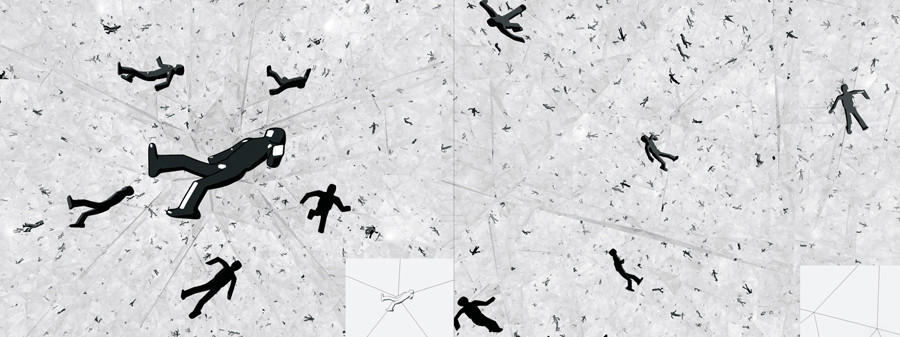## solution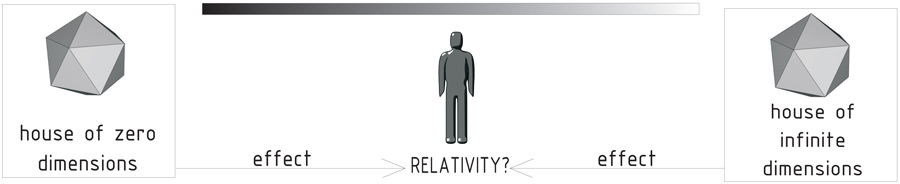Space is defined by three dimensions. Those dimensions are reflected and multiplied an infinite number of times within the icosahedron. With every reflection being slightly different than the previous one, we achieve a fractal space of infinite variety - a house of infinite dimensions.

## 3 x ∞ = ∞